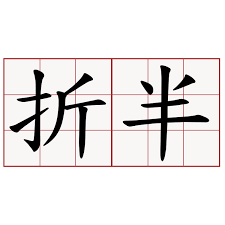# 精选算法面试 - 数组（二分查找）## 二.示例

### 2.1 查找元素位置，没有找到返回插入位置

public int searchInsert(int[] nums, int target) {    int left = 0, right = nums.length;    while (left < right)    {        int mid = left + (right - left) / 2;        if (nums[mid] >= target)        {            right = mid;        }        else        {            left = mid + 1;        }    }    return left;}

### 2.2 查找第一个元素

public int binaryByFirst(int[] a,int n,int value){    int lo=0;    int hi=n;    int mid = 0;    while (lo <= hi){        //为了数组越界        mid = lo+((hi-lo)>>1);        if(a[mid] > value){            hi = mid - 1;        }else if(a[mid] < value){            lo=mid+1;        }else{            if((mid == 0) || (a[mid - 1] != value)) return mid;            else hi = mid - 1;        }    }    return -1;}

### 2.3 查找第一个大于等于给定值的元素

public int binaryByFirstBig(int[] a,int n,int value){    int lo=0;    int hi=n;    int mid = 0;    while (lo <= hi){        //为了数组越界        mid = lo+((hi-lo)>>1);        if(a[mid] >= value){            if((mid == 0) || a[mid-1] < value){                return mid;            }else {                hi = mid -1;            }        }else {            lo = mid +1;        }    }    return -1;}

### 2.4 查找最后一个值等于给定值的元素

public int binaryByEnd(int[] a,int n,int value){    int lo=0;    int hi=n;    int mid = 0;    while (lo <= hi){        mid = lo+((hi-lo)>>1);        if(a[mid] > value){            hi = mid - 1;        }else if(a[mid] < value){            lo=mid+1;        }else{            if((mid == n) || (a[mid + 1] != value)) return mid;            else lo = mid + 1;        }    }    return -1;}

### 2.5 查找最后一个小于等于给定值的元素

public int binaryByEndBig(int[] a,int n,int value){int lo = 0;int hi = n;int mid = 0;while (lo <= hi){mid = lo+((hi-lo)>>1);if(a[mid] <= value){if(mid == n || a[mid] > value){return mid;}else{lo = mid+1;}}else {hi = mid -1;}}return -1;}## 评论